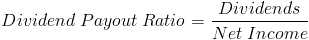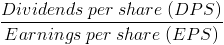# Dividend Payout RatioThe dividend payout ratio is the amount of dividends paid to stockholders relative to the amount of total net income of a company. The amount that is not paid out in dividends to stockholders is held by the company for growth. The amount that is kept by the company is called retained earnings.

Net income shown in the formula can be found on the company's income statement.

This formula is used by some when considering whether to invest in a profitable company that pays out dividends versus a profitable company that has high growth potential. In other words, this formula takes into consideration steady income versus reinvestment for possible future earnings, assuming the company has a net income.

## Alternative Formula

I.The retention ratio and the dividend payout ratio together equal 1 or 100% of net income. The premise is that whatever amount not paid in dividends is kept by the company to reinvest for expansion.

A simple example would be a company who pays out 100% of their net income in dividends. In this situation, net income would be equal to dividends. Using the formula for this example, the dividend payout ratio would be 1 or 100%. The retention ratio would be 0 or 0% as they do not retain and reinvest any of their earnings for growth. Using the alternative formula 1 - 0 would be 1.

Alternatively, a company who pays no dividends would have a 0 dividend payout ratio and a 1 retention ratio, which means that the company reinvests all of their net income for growth.

II.The dividend payout ratio formula can also be restated on a "per share" basis. If the dividend per share and earnings per share is known, the dividend payout ratio can be calculated using the same concept of dividends paid divided by earnings, or net income.

New to Finance?